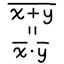#Boolean algebra laws

## Notation

The following notation is used for Boolean algebra on this page, which is the electrical engineering notation:

• False: 0
• True: 1
• NOT x: x
• x AND y: xy
• x OR y: x + y
• x XOR y: xy

The precedence from high to low is AND, XOR, OR. Examples:

• x + yz means x + (yz)
• xyz means x ⊕ (yz)
• x + yz means x + (yz)

## Basic laws

• NOT:
• 0 = 1
• 1 = 0
• AND:
• 0 ⋅ 0 = 0
• 0 ⋅ 1 = 0
• 1 ⋅ 0 = 0
• 1 ⋅ 1 = 1
• OR:
• 0 + 0 = 0
• 0 + 1 = 1
• 1 + 0 = 1
• 1 + 1 = 1
• XOR:
• 0 ⊕ 0 = 0
• 0 ⊕ 1 = 1
• 1 ⊕ 0 = 1
• 1 ⊕ 1 = 0

• AND:
• 0 ⋅ x = 0
• 1 ⋅ x = x
• OR:
• 0 + x = x
• 1 + x = 1
• XOR:
• 0 ⊕ x = x
• 1 ⊕ x = x

• NOT:
• NOT x = x
• AND:
• xx = x
• xx = 0
• OR:
• x + x = x
• x + x = 1
• XOR:
• xx = 0
• xx = 1

### XOR

XOR can be defined in terms of AND, OR, NOT:

• xy = (xy) + (xy)
• xy = (x + y) ⋅ (x + y)
• xy = (x + y) ⋅ (xy)

### Commutativity

• AND: xy = yx
• OR: x + y = y + x
• XOR: xy = yx

### Associativity

• AND: (xy) ⋅ z = x ⋅ (yz)
• OR: (x + y) + z = x + (y + z)
• XOR: (xy) ⊕ z = x ⊕ (yz)

### Distributivity

• x ⋅ (y + z) = (xy) + (xz)
• x + (yz) = (x + y) ⋅ (x + z)
• x ⋅ (yz) = (xy) ⊕ (xz)

### De Morgan’s laws

• NAND: xy = x + y
• NOR: x + y = xy

## Redundancy laws

The following laws will be proved with the basic laws. Counter-intuitively, it is sometimes necessary to complicate the formula before simplifying it.

### Absorption

• x + xy = x

Proof:
x + xy
= x ⋅ 1 + xy
= x ⋅ (1 + y)
= x ⋅ 1
= x

• x ⋅ (x + y) = x

Proof:
x ⋅ (x + y)
= (x + 0) ⋅ (x + y)
= x + (0 ⋅ y)
= x + 0
= x

### No name

• x + xy = x + y

Proof:
x + xy
= (x + x) ⋅ (x + y)
= 1 ⋅ (x + y)
= x + y

• x ⋅ (x + y) = xy

Proof:
x ⋅ (x + y)
= xx + xy
= 0 + xy
= xy

• xy + xy = x

Proof:
xy + xy
= x ⋅ (y + y)
= x ⋅ 1
= x

• (x + y) ⋅ (x + y) = x

Proof:
(x + y) ⋅ (x + y)
= x + (yy)
= x + 0
= x

### Consensus

• xy + xz + yz = xy + xz

Proof:
xy + xz + yz
= xy + xz + 1 ⋅ yz
= xy + xz + (x + x) ⋅ yz
= xy + xz + xyz + xyz
= xy + xyz + xz + xyz
= xy ⋅ 1 + xyz + x ⋅ 1 ⋅ z + xyz
= xy ⋅ (1 + z) + xz ⋅ (1 + y)
= xy ⋅ 1 + xz ⋅ 1
= xy + xz

• (x + y) ⋅ (x + z) ⋅ (y + z) = (x + y) ⋅ (x + z)

Proof:
(x + y) ⋅ (x + z) ⋅ (y + z)
= (x + y) ⋅ (x + z) ⋅ (0 + y + z)
= (x + y) ⋅ (x + z) ⋅ (xx + y + z)
= (x + y) ⋅ (x + z) ⋅ (x + y + z) ⋅ (x + y + z)
= (x + y) ⋅ (x + y + z) ⋅ (x + z) ⋅ (x + y + z)
= (x + y + 0) ⋅ (x + y + z) ⋅ (x + 0 + z) ⋅ (x + y + z)
= (x + y + 0 ⋅ z) ⋅ (x + z + 0 ⋅ y)
= (x + y + 0) ⋅ (x + z + 0)
= (x + y) ⋅ (x + z)